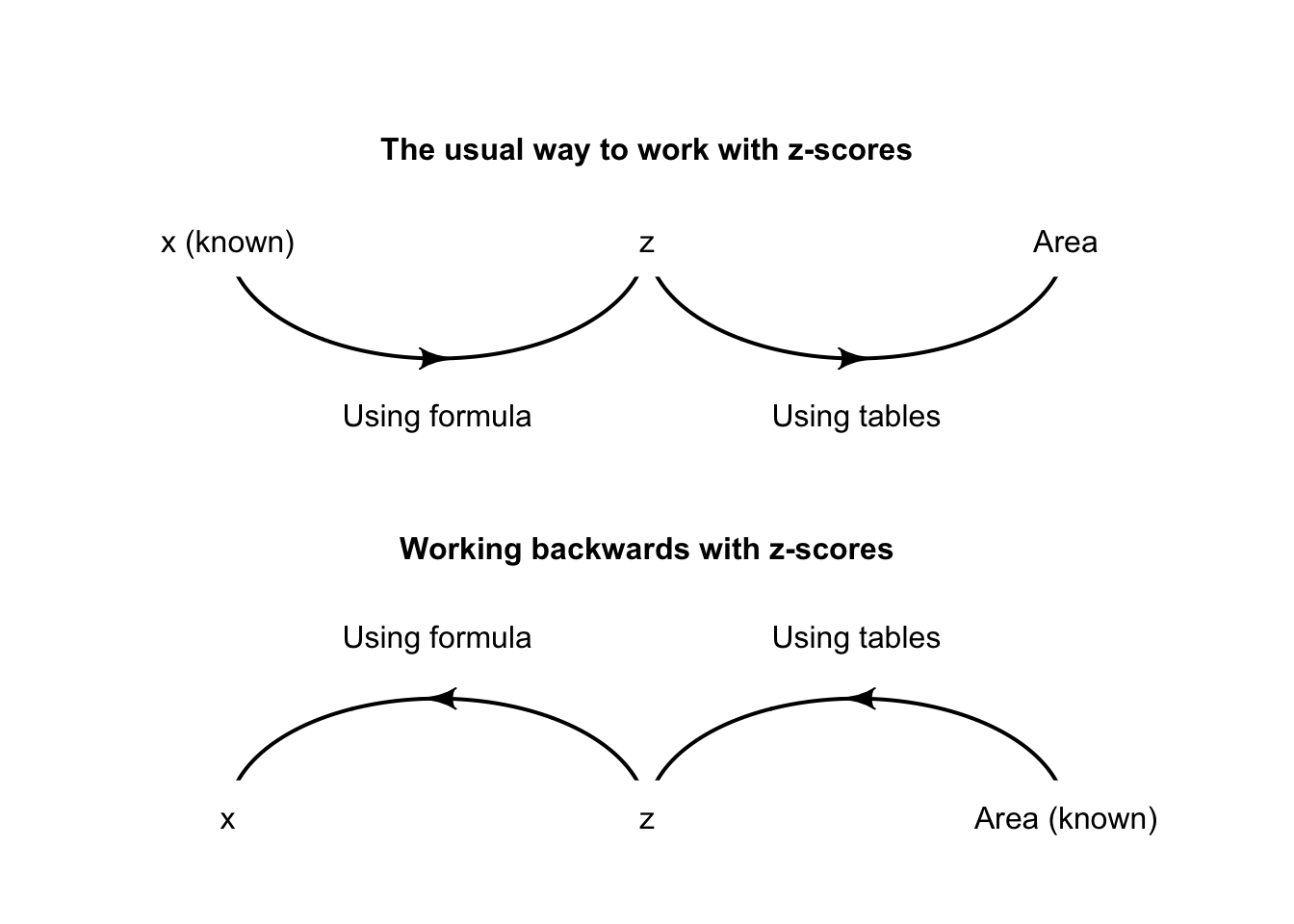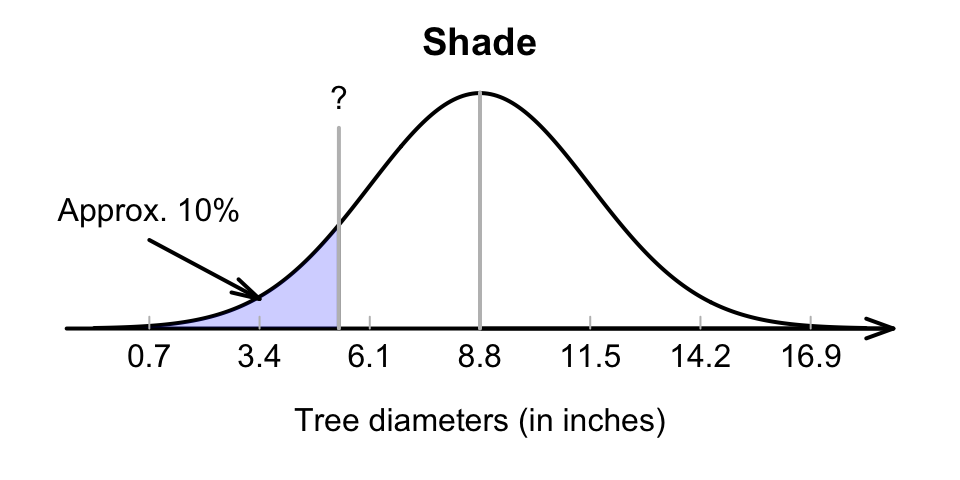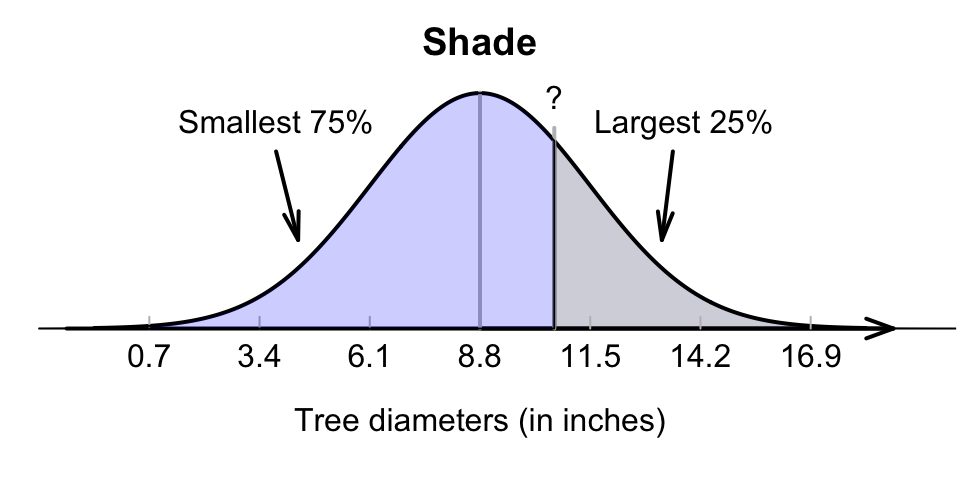## 17.9 Unstandardising: Working backwards

Using the model for tree diameters in Example 17.7 again, suppose now the diameters of the smallest 10% of trees needs to be identified. What are these diameters?

Example 17.9 (Normal distributions backwards) Consider again the trees study. The tree diameters can be modelled with

• a normal distribution; with
• a mean of $$\mu=8.8$$ inches; and
• a standard deviation of $$\sigma=2.7$$ inches.
Identify the diameters of the smallest 10% of trees,

This is a different problem than before; previously, the tree diameter was known, so a $$z$$-score could be computed, and hence a probability (Fig. 17.11, top panel). This time, the probability is known, and a tree diameter is sought. That is, working ‘backwards’ is needed (Fig. 17.11, bottom panel), so the $$z$$-tables need to be used ‘backwards’ too.FIGURE 17.11: Working with $$z$$-scores

### 17.9.1 Using the hard-copy tables

When the $$z$$ scores (in the margins of the tables were known, the areas were found in the body of the table. If the area (or probability) is known (found in the body of the table), the corresponding $$z$$-score can be found (in the margins of the table), and hence the observation $$x$$; see the animation below. The closest area to 10% in the tables is 0.1003, or 10.03%.

To identify the diameters of the smallest 10% of trees, the $$z$$-score that has an area to the left of 10% (or 0.10) need to be found (at least, as close as possible to 0.10).

### 17.9.2 Using the online tables

When the area (or probability) is known, special online tables can be used (Appendix B.3). In these tables, enter the area to the left in search box under Area.to.left, and the corresponding $$z$$-scores appears under the z.score column (see the animation below).

Using either the hard-copy or online tables, the appropriate $$z$$-value is $$1.28$$ standard deviations below the mean (Fig. 17.12). Then, the $$z$$-score can be converted to an observation value $$x$$ using the unstandardising formula6:

$x = \mu + z\sigma.$ Using this unstandardising formula:

\begin{align*} x &= \mu + (z\times\sigma) \\ &= 8.8 + (-1.28 \times 2.7) = 5.344; \end{align*} that is, about 10% of trees have diameters less than about 5.3 inches.FIGURE 17.12: Tree diameters: The smallest 10%

Definition 17.2 (Unstandardizing formula) When the $$z$$-score is known, the corresponding value of the observation $$x$$ is

$\begin{equation} x = \mu + z\sigma. \tag{17.2} \end{equation}$ This is called the unstandardising formula.
Think 17.2 (Normal tables backwards) Ball bearings labelled as “50mm bearings” actually have diameters that follow a normal distribution with mean 50mm and standard deviation 0.1mm. The smallest 15% of bearings are too small for sale. What size bearings cannot be sold?
The closest area from the tables is 0.1492, corresponding to $$z = -1.04$$. Using the unstandardising formula, $$x = 50 + (-1.04\times 0.1)$$, or 49.896: Bearings less than about 49.9 mm in diameter cannot be sold.

Example 17.10 (Normal distributions backwards) Using the model for tree diameters in Example 17.7 again, suppose now the diameters of the largest 25% of trees needs to be identified. What are these diameters?

The tree diameters can be modelled with

• a normal distribution; with
• a mean of $$\mu=8.8$$ inches; and
• a standard deviation of $$\sigma=2.7$$ inches.

Again, we need to work ‘backwards’ (Fig. 17.13, bottom panel), so the $$z$$-tables need to be used ‘backwards’ too. The largest 25% implies large trees, so we would expect a diameter larger than the mean.

Using a diagram is important (Fig. 17.13): the tables work with the area to the left of the value of interest, which is 75%.

Using either the hard-copy or online tables, the appropriate $$z$$-value is $$z = 0.674$$. Then, the $$z$$-score can be converted to an observation value $$x$$ using the unstandardising formula:

\begin{align*} x &= \mu + (z\times\sigma) \\ &= 8.8 + (0.674 \times 2.7) = 10.621; \end{align*} that is, about 25% of trees have diameters larger than about 10.6 inches.FIGURE 17.13: Tree diameters: The largest 25% is the same as the smallest 75%

### References

Aedo-Ortiz DM, Olsen ED, Kellogg LD. Simulating a harvester-forwarder softwood thinning: A software evaluation. Forest Products Journal. 1997;47(5):36–41.

1. This is found by re-arranging Equation (17.1).↩︎